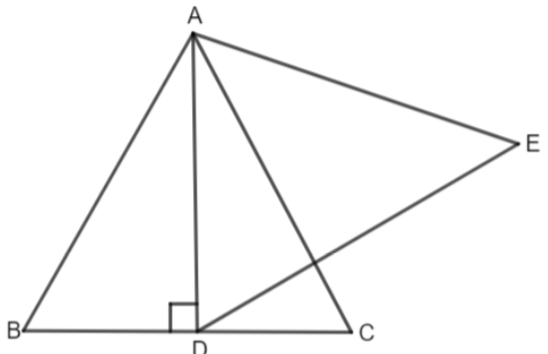QuestionAnswers

# AD is an altitude of an equilateral triangle ABC. On AD as the base, another equilateral triangle ADE is constructed, then area (Triangle ADE): area (Triangle ABC) = 3:4.(a) True(b) FalseVerified
58.8k+ views
Hint: As we have both the triangles as equilateral triangles, so they are similar. We know that if triangle ABC is congruent to triangle PQR, then, $\dfrac{Ar\left( \Delta ABC \right)}{Ar\left( \Delta PQR \right)}={{\left( \dfrac{AB}{PQ} \right)}^{2}}={{\left( \dfrac{BC}{QR} \right)}^{2}}={{\left( \dfrac{AC}{PR} \right)}^{2}}.$ So, we compare the sides to find the ratio of the area between triangle ADE and triangle ABC.

Complete step-by-step solution:
We are given that ABC is an equilateral triangle. Let us consider its side as ‘a’. So, we get,
$AB=BC=AC=a......\left( i \right)$
AD is the altitude through while we will construct another triangle ADE.We know that in triangle ABC, altitude is the perpendicular bisector. So, we have,
$BD=DC......\left( ii \right)$
$BC=BD+DC$
From (ii), we have BD = DC, so we get,
$\Rightarrow BC=BD+BD$
$\Rightarrow BC=2BD$
So, we get,
$\Rightarrow \dfrac{BC}{2}=BD$
Noe from (i), we get,
$BD=\dfrac{a}{2}....\left( iii \right)$
As AD is a perpendicular bisector, we get triangle ABD as right triangle. Now, applying Pythagoras theorem in triangle ABD which says
$A{{D}^{2}}+B{{D}^{2}}=A{{B}^{2}}$
From here, we get,
$A{{D}^{2}}=A{{B}^{2}}-B{{D}^{2}}$
Using the value of (i) and (iii), we get,
$A{{D}^{2}}={{a}^{2}}-\dfrac{{{a}^{2}}}{4}$
$\Rightarrow A{{D}^{2}}=\dfrac{4{{a}^{2}}-{{a}^{2}}}{4}$
$\Rightarrow A{{D}^{2}}=\dfrac{3{{a}^{2}}}{4}$
Now, canceling square on both the sides, we get,
$AD=\dfrac{\sqrt{3}a}{2}.....\left( iv \right)$
So, we get the length of the sides of the triangle ADC as $AD=\dfrac{\sqrt{3}a}{2}.$
As triangle ABC and triangle ADE are both equilateral triangles, this means that both the triangles have all the angles as ${{60}^{\circ }}.$ Therefore, by AAA similarity criteria, they are similar.
So,
$\dfrac{Ar\left( \Delta ADE \right)}{Ar\left( \Delta ABC \right)}={{\left( \dfrac{AD}{AB} \right)}^{2}}$
Using (i) and (iv), we get,
$\Rightarrow \dfrac{Ar\left( \Delta ADE \right)}{Ar\left( \Delta ABC \right)}=\dfrac{{{\left( \dfrac{\sqrt{3}{a}}{2} \right)}^{2}}}{{{a}^{2}}}$
$\Rightarrow \dfrac{Ar\left( \Delta ADE \right)}{Ar\left( \Delta ABC \right)}=\dfrac{\dfrac{3{{a}^{2}}}{4}}{{{a}^{2}}}$
$\Rightarrow \dfrac{Ar\left( \Delta ADE \right)}{Ar\left( \Delta ABC \right)}=\dfrac{3{{a}^{2}}}{4{{a}^{2}}}$
$\Rightarrow \dfrac{Ar\left( \Delta ADE \right)}{Ar\left( \Delta ABC \right)}=\dfrac{3}{4}$
So, we get,
$\dfrac{Ar\left( \Delta ADE \right)}{Ar\left( \Delta ABC \right)}=\dfrac{3}{4}$
Hence, option (a) is the right answer.

Note: The alternate method to find AD, i.e the altitude is as follows. We know that the area of the equilateral triangle is also given as
$\text{Area}=\dfrac{\sqrt{3}}{4}\times {{\left( \text{side} \right)}^{2}}$
In triangle ABC with base BC and altitude AD, we get,
$\text{Area of }\Delta \text{ABC}=\dfrac{1}{2}\times BC\times AD$
Also,
$\text{Area of }\Delta ABC=\dfrac{\sqrt{3}}{4}{{\left( \text{side} \right)}^{2}}$
$\Rightarrow \text{Area of }\Delta ABC=\dfrac{\sqrt{3}}{4}\times B{{C}^{2}}$
So comparing both, we get,
$\dfrac{1}{2}\times BC\times AD=\dfrac{\sqrt{3}}{4}\times B{{C}^{2}}$
$\Rightarrow AD=\dfrac{\sqrt{3}}{4}\times B{{C}^{2}}\times \dfrac{2}{BC}$
$\Rightarrow AD=\dfrac{\sqrt{3}}{2}\times BC$
$\Rightarrow AD=\dfrac{\sqrt{3}}{2}a$